# SQL AVG() function

## AVG() function

SQL AVG() function calculates the average value of a column of numeric type. It returns the average of all non NULL values

Syntax:

```AVG ([ALL | DISTINCT] expression )
```

DBMS Support: COUNT() function

 DBMS Command MySQL Supported PostgreSQL Supported SQL Server Supported Oracle Supported

DB2 and Oracle Syntax:

```AVG ([ALL | DISTINCT] expression ) OVER (window_clause)
```

Parameters:

Name Description
ALL Applies to all values.
DISTINCT Return the sum of unique values.
expression Expression made up of a single constant, variable, scalar function, or column name. The expression is an expression of the exact numeric or approximate numeric data type category, except for the bit data type. Aggregate functions and subqueries are not permitted.

Syntax diagram - AVERAGE() functionExample:

To get the data the average of 'advance_amount' from the 'orders' table, the following SQL statement can be used :

``````SELECT AVG( advance_amount )
FROM orders;``````

Sample table: orders

Output:

```AVG(ADVANCE_AMOUNT)
-------------------
629.166667
```

Pictorial Presentation: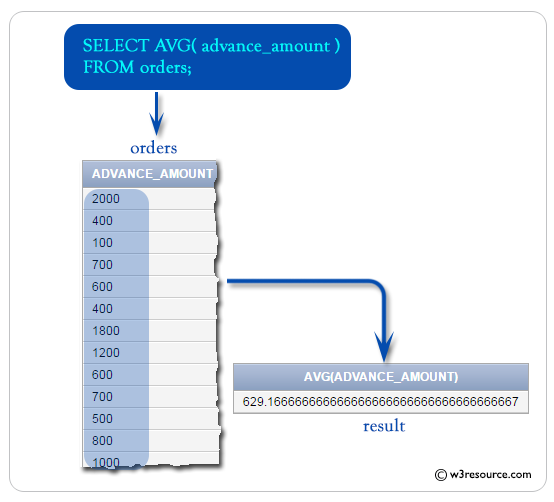## SQL AVG() with null

To get the data of 'agent_code', sum of 'opening_amt', number of customer for each agent, and 'receive_amt' from the 'customer' table, the following SQL statement can be used :

``````SELECT agent_code, SUM (opening_amt),
COUNT(*), ROUND(SUM (opening_amt) /COUNT(*)) AS MYAVG,
ROUND(AVG (opening_amt)) AS SQLAVG
FROM customer
GROUP BY agent_code;``````

Sample table : customer

Output:

```AGENT_CODE SUM(OPENING_AMT)   COUNT(*)      MYAVG     SQLAVG
---------- ---------------- ---------- ---------- ----------
A002                  22000          3       7333       7333
A004                  25000          3       8333       8333
A007                  16000          2       8000       8000
A009                   6000          1       6000       6000
A011                   5000          1       5000       5000
A012                   5000          1       5000       5000
A010                  22000          3       7333       7333
A001                   8000          1       8000       8000
A008                  13000          3       4333       4333
A006                   8000          2       4000       4000
A005                  19000          3       6333       6333
A003                  12000          2       6000       6000
```

## SQL AVG() with where

To get the average of 'advance_amount' from the 'orders' table with the following conditions -

1. 'ord_amount' must be more than 1000,

2. and 'ord_amount' must be up to 4500,

the following SQL statement can be used :

``````SELECT AVG( advance_amount)
FROM orders
WHERE ord_amount>1000 AND ord_amount <= 4500;``````

Sample table : orders

Relational Algebra Expression: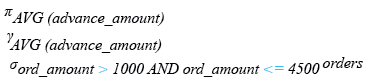Relational Algebra Tree: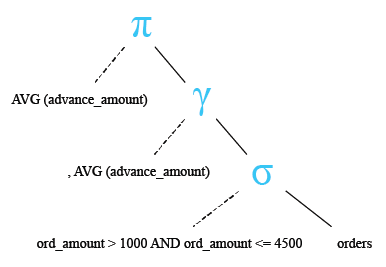Output:

```AVG(ADVANCE_AMOUNT)
-------------------
800
```

Pictorial Presentation: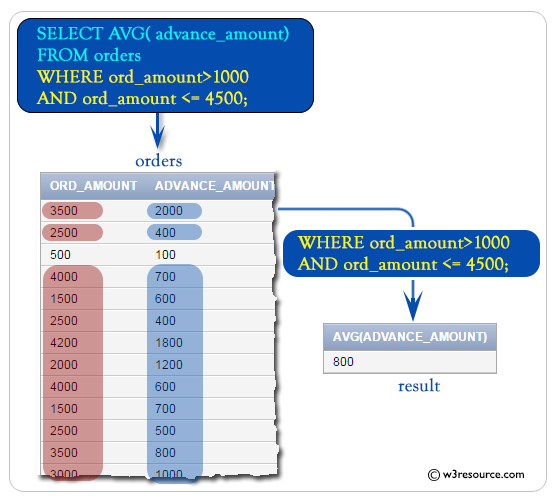## SQL AVG() with SUM()

To get the sum of 'advance_amount' and average of 'advance_amount' from the 'orders' table, the following SQL statement can be used :

``````
FROM orders;``````

Sample table : orders

Relational Algebra Expression: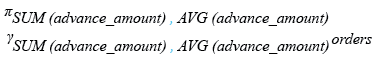Relational Algebra Tree: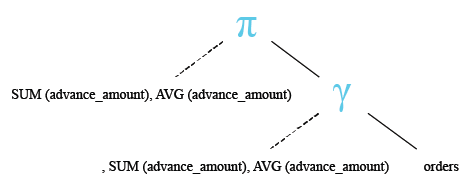Output:

```SUM(ADVANCE_AMOUNT) AVG(ADVANCE_AMOUNT)
------------------- -------------------
22650          629.166667
```

Note: Outputs of the said SQL statement shown here is taken by using Oracle Database 10g Express Edition

Here is a slide presentation of all aggregate functions.

Check out our 1000+ SQL Exercises with solution and explanation to improve your skills.

﻿

## SQL: Tips of the Day

Is SQL syntax case sensitive?

The SQL Keywords are case-insensitive (SELECT, FROM, WHERE, etc), but are often written in all caps. However in some setups table and column names are case-sensitive. MySQL has a configuration option to enable/disable it. Usually case-sensitive table and column names are the default on Linux MySQL and case-insensitive used to be the default on Windows, but now the installer asked about this during setup. For MSSQL it is a function of the database's collation setting.

Ref: https://bit.ly/3R2iyNZ

We are closing our Disqus commenting system for some maintenanace issues. You may write to us at reach[at]yahoo[dot]com or visit us at Facebook